### Home > PC > Chapter 12 > Lesson 12.2.2 > Problem12-55

12-55.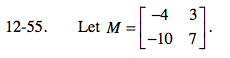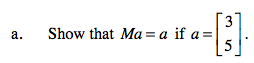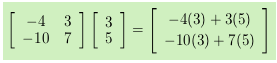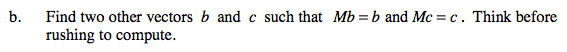In general,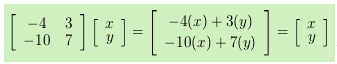Set up a system of equations and solve for y in terms of x.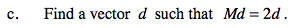For parts (c), (d), and (e), follow the steps in part (b).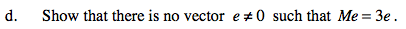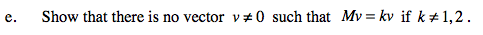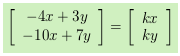Solving for x in the top equation and y in the bottom equation: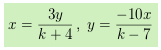By substitution: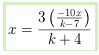x(k + 4)(k − 7) = −30x

Since we have assumed x ≠ 0, divide by x and solve for k.